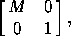# 10.2 Formulas for Symmetries in Homogeneous Coordinates

All isometries of space can be expressed in homogeneous coordinates in terms of multiplication by a matrix. As in the case of plane isometries (Section 2.2), this means that the successive application of transformations reduces to matrix multiplication. (In the formulas below,is the 4×4 projective matrix obtained from the 3×3 matrix M by adding a row and a column as stated.)

Translation by (x,y,z):Rotation through the origin:where M is given in (10.1.1) or (10.1.2) , as the case may be.

Reflection in a plane through the origin:where M is given in (10.1.3) .

From this one can deduce all other transformations, as in the case of plane transformations.

Next: 11 Other Transformations of Space
Up: 10 Space Symmetries or Isometries
Previous: 10.1 Formulas for Symmetries in Cartesian CoordinatesThe Geometry Center Home Page

Silvio Levy
Wed Oct 4 16:41:25 PDT 1995

This document is excerpted from the 30th Edition of the CRC Standard Mathematical Tables and Formulas (CRC Press). Unauthorized duplication is forbidden.